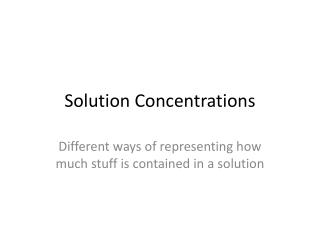DownloadDownload PresentationSolution Concentrations

# Solution Concentrations

Download Presentation## Solution Concentrations

- - - - - - - - - - - - - - - - - - - - - - - - - - - E N D - - - - - - - - - - - - - - - - - - - - - - - - - - -
##### Presentation Transcript

1. Solution Concentrations Different ways of representing how much stuff is contained in a solution

2. Solution Components • Solution: a homogeneous mixture of two or more substances in a single phase • Atoms, molecules, or ions are thoroughly mixed so that the substance has a consistent composition and properties • Solute: The substance being dissolved • USUALLY the smaller amount • The particles of solute are so small that they can not be seen • Solvent: The substance doing the dissolving

3. Some Commonly Used Ways of Representing Concentration • Molality • Moles of solute per kilogram of solvent • Percent by Volume • Percent by Mass • Parts per Million • Parts per Billion • Parts per Trillion

4. Molarity • Number of moles of solute per liter of solution

5. Perform the following calculations… • What is the molarity of a solution prepared by dissolving 37.94 g of potassium hydroxide to produce a 500.0 mL solution? • What is the molarity of a 3500.0 mL solution that contains 141.6 g of citric acid (C3H5O(COOH)3)? • How much sodium hydroxide would be needed to produce 750.0 mL of a 6.00 M solution? • How many grams of K2Cr2O7 are needed to make 250.0 mL of a 0.125M solution?

6. Preparing Solutions by Dilution • In many cases, solutions are sold in high concentrations and diluted with distilled water (or other solvents) to produce a solution with a desired concentration • Any ideas why? • When this is the case, the number of moles is the quantity that will determine the volumes needed…

7. Perform the following calculation • Calculate the amount of a 12.0 M hydrochloric acid solution (HCl) that is needed to produce 500.0 mL of a 2.00 M HCl solution. • Determine the moles of solute required for the desired solution • Use the SAME NUMBER OF MOLES to calculate the volume of the concentrated solution that would provide this amount • Make up the volume difference with distilled water (or other specified solvent, if you are actually making the solution)

8. Perform the following calculations… • What volume of a 6.77 M nitric acid (HNO3) solution to produce a 250.0 mL of a 1.50 M solution? • What is the molarity of a solution that is made by diluting 50.00 ml of a 4.74 M HCl solution to 250.0 mL? • How much final solution would be made by adding water to 1.19 mL of a 8.00 M acetic acid solution (CH3COOH) to produce a final molarity of 1.50 M?

9. Perform the following calculations… • What is the final molarity of an ammonium chloride solution produced by diluting 50.00 mL of a 3.79 M solution to 2.00 L? • A 3.8 M solution of iron (II) sulfate is diluted to eight times its original volume. What is the new molarity of the solution?